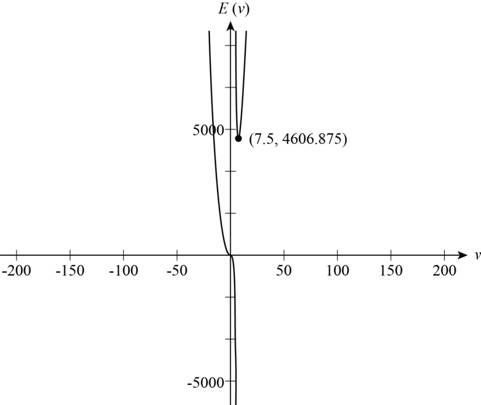# The value of v which minimizes the total energy required to swim a fixed distance.### Precalculus: Mathematics for Calcu...

6th Edition
Stewart + 5 others
Publisher: Cengage Learning
ISBN: 9780840068071### Precalculus: Mathematics for Calcu...

6th Edition
Stewart + 5 others
Publisher: Cengage Learning
ISBN: 9780840068071

#### Solutions

Chapter 2.3, Problem 52E
To determine

## The value of v which minimizes the total energy required to swim a fixed distance.

Expert Solution

The value of v which minimizes the total energy is 7.5mi/h.

### Explanation of Solution

Given:

The total energy required to swim a distance of 10 mi against a current of 5 mi/h is given by,

E(v)=2.73v310v5

Calculation:

Use a graphing calculator to draw the graph of the function E with respect to v as shown in Figure 1.Figure (1)

Locate the point at which local minimum occurs to find the value of v which minimizes the total energy.

The value E(b) is local minimum value if E(b)E(v) when b is near v.

From Figure (1), it is noticed that there is one local minimum between v=0 and v=10 .

Point (7.5,4606.875) is the lowest point. This point is the point of local minimum.

The value 4606.875 is minimum for v=7.5 .

Therefore, the value of v which minimizes the total energy is 7.5mi/h.

### Have a homework question?

Subscribe to bartleby learn! Ask subject matter experts 30 homework questions each month. Plus, you’ll have access to millions of step-by-step textbook answers!